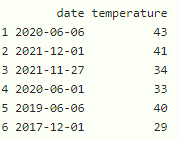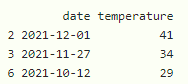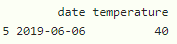Open in App
Not now

# Subset DataFrame Between Two Dates in R

• Last Updated : 11 Oct, 2021

In this article, we are going to get the data between two dates in dataframe in R Programming Language.

We can create a column to store date using as.Date() function

Syntax:

as.Date(‘yyyy-mm-dd’)

Example: Creating dataframe

## R

 `# create a dataframe with 6 rows and 2 columns``# one column is of 6 dates with date type as``# year-month-day format``# second is temperature degrees``data = ``data.frame``(date=``c``(``as.Date``(``'2020-6-6'``),``                         ``as.Date``(``'2021-12-1'``),``                         ``as.Date``(``'2021-11-27'``),``                         ``as.Date``(``'2020-6-1'``),``                         ``as.Date``(``'2019-6-6'``),``                         ``as.Date``(``'2017-12-1'``)),``                  ``temperature=``c``(43, 41, 34, 33, 40, 29))` `# display``print``(data)`

Output:We are going to subset the data between date ranges using logical operators. Thus, we need less than(<), greater than(>), and the and(&) operator.

Syntax:

dataframe[dataframe\$date_column> “start_date” &  dataframe\$date_column < “end_date”, ]

where,

• dataframe is the input dataframe
• date_column is the date column in the dataframe
• date is the date specified to get the data from  start_date to end_date

Example: R program to get the data from the given date ranges

## R

 `# create a dataframe with 6 rows and 2 columns``# one column is of 6 dates with date type as``# year-month-day format``# second is temperature degrees``data=``data.frame``(date=``c``(``as.Date``(``'2020-6-6'``),``                       ``as.Date``(``'2021-12-1'``),``                       ``as.Date``(``'2021-11-27'``),``                       ``as.Date``(``'2020-6-1'``),``                       ``as.Date``(``'2019-6-6'``),``                       ``as.Date``(``'2021-10-12'``)),``                ``temperature=``c``(43,41,34,33,40,29))` `# subset the dataframe from 1 st january 2021``# to 1 st October 2022``print``(data[data\$date > ``"2021-01-01"` `&   data\$date < ``"2022-10-01"``, ])`

Output:Example: R program to get the data from the given date ranges

## R

 `# create a dataframe with 6 rows and 2 columns``# one column is of 6 dates with date type as``# year-month-day format``# second is temperature degrees``data=``data.frame``(date=``c``(``as.Date``(``'2020-6-6'``),``                       ``as.Date``(``'2021-12-1'``),``                       ``as.Date``(``'2021-11-27'``),``                       ``as.Date``(``'2020-6-1'``),``                       ``as.Date``(``'2019-6-6'``),``                       ``as.Date``(``'2017-10-12'``)),``                ``temperature=``c``(43,41,34,33,40,29))` `# subset the dataframe from 11th november 2017``# to 1 st October 2019``print``(data[data\$date > ``"2017-11-11"` `&   data\$date < ``"2019-10-01"``, ])`

Output:My Personal Notes arrow_drop_up